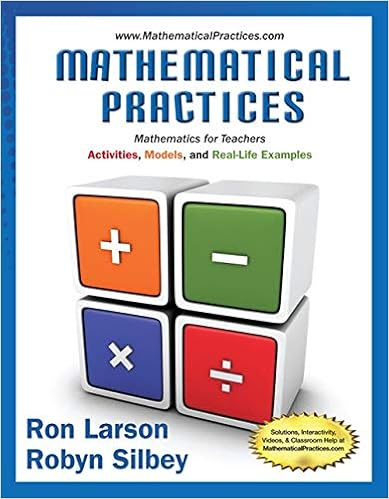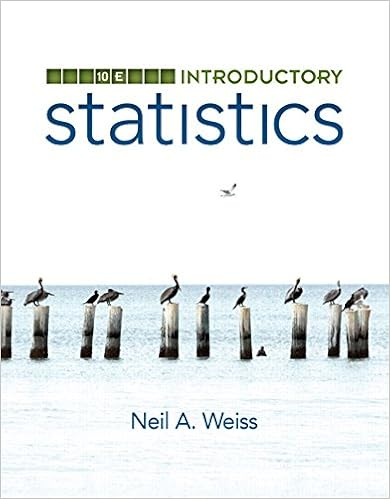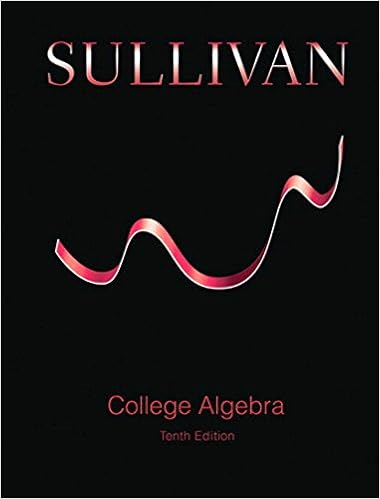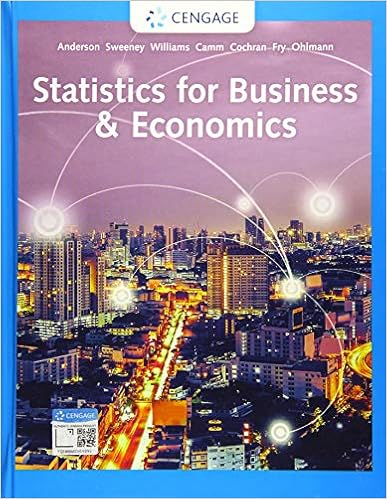• Assignment
• 144
• 100% (4) 4 out of 4 people found this document helpful

This preview shows page 1 - 3 out of 144 pages.

The preview shows page 1 - 3 out of 144 pages.
11PARAMETRIC EQUATIONS,POLAR COORDINATES, ANDCONIC SECTIONS11.1Parametric Equations(LT Section 12.1)Preliminary Questions1.Describe the shape of the curvex=3 cost,y=3 sint.
2.How doesx=4+3 cost,y=5+3 sintdiffer from the curve in the previous question?
3.What is the maximum height of a particle whose path has parametric equationsx=t9,y=4t2?
4.Can the parametric curve(t,sint)be represented as a graphy=f(x)? What about(sint,t)?
5. (a)Describe the path of an ant that is crawling along the plane according toc1(t)=(f(t),f(t)), wheref(t)is an increasing function.(b)Compare that path to the path of a second ant crawling according toc2(t)=f(2t),f(2t)).solution
6.Find three different parametrizations of the graph ofy=x3.
1501
##### We have textbook solutions for you!The document you are viewing contains questions related to this textbook.
Chapter 8 / Exercise 8
Mathematical Practices, Mathematics for Teachers: Activities, Models, and Real-Life Examples
LarsonExpert VerifiedBrowse all Textbook Solutions
1502C H A P T E R11PARAMETRIC EQUATIONS, POLAR COORDINATES, AND CONIC SECTIONS(LT CHAPTER 12)7.Match the derivatives with a verbal description:(a)dxdt(b)dydt(c)dydx(i)Slope of the tangent line to the curve(ii)Vertical rate of change with respect to time(iii)Horizontal rate of change with respect to timesolution(a)The derivativedxdtis the horizontal rate of change with respect to time.

Course Hero member to access this document

Course Hero member to access this document

End of preview. Want to read all 144 pages?

Course Hero member to access this document

Term
Winter
Professor
Justin
Tags
Parametric equation, Conic section, Vector valued function
##### We have textbook solutions for you!
The document you are viewing contains questions related to this textbook.The document you are viewing contains questions related to this textbook.
Chapter 8 / Exercise 8
Mathematical Practices, Mathematics for Teachers: Activities, Models, and Real-Life Examples
LarsonExpert Verified
•••